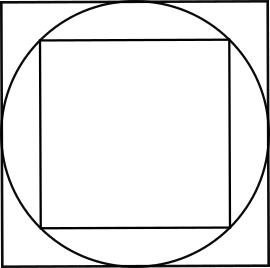#### You may also like### Dividing the Field

A farmer has a field which is the shape of a trapezium as illustrated below. To increase his profits he wishes to grow two different crops. To do this he would like to divide the field into two trapeziums each of equal area. How could he do this?### Two Circles

Draw two circles, each of radius 1 unit, so that each circle goes through the centre of the other one. What is the area of the overlap?### Star Gazing

Find the ratio of the outer shaded area to the inner area for a six pointed star and an eight pointed star.

# Square in a Circle in a Square

##### Age 14 to 16 Short Challenge Level:

In the diagram, the circle touches every edge of the larger square and every corner of the smaller square.What is the ratio of the area of the smaller square to the area of the larger square?

This problem is adapted from the World Mathematics Championships
You can find more short problems, arranged by curriculum topic, in our short problems collection.